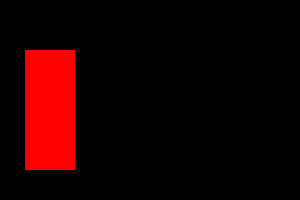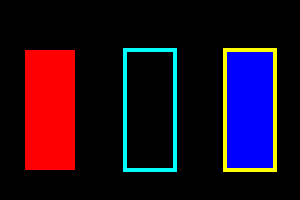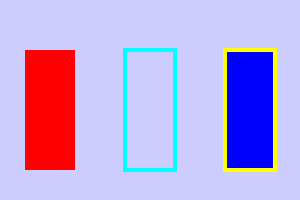# Basic drawing in Pycairo

By Martin McBride, 2018-04-14
Tags: imagesurface context rectangle
Categories: pycairoIn this article we will learn how the basics of drawing in Pycairo. We will also look at how to set the scale your drawing.

It is assumed that you have already installed Pycairo on your system.

## Making an image with Pycairo

There are 4 basic steps to creating an image:

• Create a Pycairo surface to hold your drawing
• Create a Pycairo context that you use to draw with
• Draw your shapes using the methods of the context object
• Save the surface to file

Here is how we create a surface:

surface = cairo.ImageSurface(cairo.FORMAT_RGB24, 300, 200)


This creates an ImageSurface (a type of Surface that is used to create PNG images). It is set to use RGB data, and given a width of 100 pixels and a height of 200 pixels.

Getting the context is easy:

ctx = cairo.Context(surface)


Next we will draw a rectangle:

ctx.rectangle(25, 50, 50, 120)
ctx.set_source_rgb(1, 0, 0)
ctx.fill()


First we define a rectangular path. A path defines a shape but doesn't actually draw it (that comes next). The rectangle function takes 4 parameters:

ctx.rectangle(x, y, width, height)


x and y set the position of the top left corner of the rectangle, relative to the top left corner of the image. The width and height set the size of the rectangle. By default these are all measured in pixels.

set_source_rgb takes 3 values in the range 0.0 to 1.0. These values specify the red, green and blue values of the colour that will be used for the next drawing operation. In this case (1, 0, 0) gives pure red.

Next, fill fills the current path (the rectangle) with the current colour (red). fill also clears the current path.

Finally we save our image as a PNG file:

surface.write_to_png('rectangle.png')


Here is the image. The default background is black (we will change that soon), our code created the red rectangle:Here is the full code:

import cairo

surface = cairo.ImageSurface(cairo.FORMAT_RGB24, 300, 200)
ctx = cairo.Context(surface)

ctx.rectangle(25, 50, 50, 120)
ctx.set_source_rgb(1, 0, 0)
ctx.fill()

surface.write_to_png('rectangle.png')


## Drawing the outline

Instead of filling a path, we can draw a line around it (in computer graphics this is called stroking). Or we can do both - fill it and outline it. Here is the image we are going to create:The left (red) rectangle is the one we created before. The middle rectangle is outlined in cyan. Here is how we do it:

ctx.rectangle(125, 50, 50, 120)
ctx.set_source_rgb(0, 1, 1)
ctx.set_line_width(4)
ctx.stroke()


The rectangle function has a different x value (125) so the rectangle is drawn in a different place. We also set_source_rgb to a different colour, (0, 1, 1) which is cyan.

Before drawing a line, we need to call set_line_width to say how wide the line shound be. Then we use stroke to outline the rectangle. The rectangle isn't filled in, so the black background shows through.

Here is how we fill and stroke a shape:

ctx.rectangle(225, 50, 50, 120)
ctx.set_source_rgb(0, 0, 1)
ctx.fill_preserve()
ctx.set_source_rgb(1, 1, 0)
ctx.set_line_width(4)
ctx.stroke()


We have, again, changed the rectangle position and colours - blue for the fill and two for the outline. Then we fill and stroke the shape as two separate operations, in a similar way to the previous two rectangles.

There is just one small difference. As mentioned above, calling fill or stroke deletes the current path. So if we called fill to fill the recatngle and the called stroke to outline it, the stroke wouldn't appear - the rectangle path has been deleted, so there is nothing to stroke (that isn't an error, the stroke function would just do nothing).

To get around this, we use fill_preserve, which fills the path without deleting it.

Here is the full code:

import cairo

surface = cairo.ImageSurface(cairo.FORMAT_RGB24, 300, 200)
ctx = cairo.Context(surface)

ctx.rectangle(25, 50, 50, 120)
ctx.set_source_rgb(1, 0, 0)
ctx.fill()

ctx.rectangle(125, 50, 50, 120)
ctx.set_source_rgb(0, 1, 1)
ctx.set_line_width(4)
ctx.stroke()

ctx.rectangle(225, 50, 50, 120)
ctx.set_source_rgb(0, 0, 1)
ctx.fill_preserve()
ctx.set_source_rgb(1, 1, 0)
ctx.set_line_width(4)
ctx.stroke()

surface.write_to_png('3rectangles.png')


## Initialising the context

At this stage it is worth looking at the initialisation code again, to add a couple of useful features.

One useful thing is to scale the page. Up until now everything has been scaled in pixels. It can be more intuitive to measure the page in "units" of our own choosing. You could think of them as inches or cm if it is a picture, or kilometres if you are drawing a map, or anything you want. We will imagine they are inches and create a small (2 by 3 inch) image.

We then need to decide how our units relate to pixels. Lets say 1 unit equals 100 pixels. This makes our image 300 by 200 pixels. Here is the scaling code:

WIDTH = 3
HEIGHT = 2
PIXEL_SCALE = 100

surface = cairo.ImageSurface(cairo.FORMAT_RGB24,
WIDTH*PIXEL_SCALE,
HEIGHT*PIXEL_SCALE)
ctx = cairo.Context(surface)
ctx.scale(PIXEL_SCALE, PIXEL_SCALE)


Here, the surface size is defined by the pixel size (the pixel width isn the WIDTH times the PIXEL_SCALE). Then we use the scale function to scale the Pycairo coordinated by PIXEL_SCALE so now everything is measured in our custom units. We must adjust our drawing code to take account of that (all our coordinate values need to be 100 times smaller). Here is how we draw the first rectangle:

ctx.rectangle(0.25, 0.5, 0.5, 1.2)
ctx.set_source_rgb(1, 0, 0)
ctx.fill()


The other thing we might want to do is set the background colour - we won't usually want it to be black. We can do this by drawing a rectangle the full size of the page and filling it with our chosen colour. This code uses light blue, but you might often prefer white:

ctx.rectangle(0, 0, WIDTH, HEIGHT)
ctx.set_source_rgb(0.8, 0.8, 1)
ctx.fill()The background must be drawn first, before you draw anything else. Here is the full code, with scaling and background:

import cairo

WIDTH = 3
HEIGHT = 2
PIXEL_SCALE = 100

surface = cairo.ImageSurface(cairo.FORMAT_RGB24,
WIDTH*PIXEL_SCALE,
HEIGHT*PIXEL_SCALE)
ctx = cairo.Context(surface)
ctx.scale(PIXEL_SCALE, PIXEL_SCALE)

ctx.rectangle(0, 0, WIDTH, HEIGHT)
ctx.set_source_rgb(0.8, 0.8, 1)
ctx.fill()

ctx.rectangle(0.25, 0.5, 0.5, 1.2)
ctx.set_source_rgb(1, 0, 0)
ctx.fill()

ctx.rectangle(1.25, 0.5, 0.5, 1.2)
ctx.set_source_rgb(0, 1, 1)
ctx.set_line_width(0.04)
ctx.stroke()

ctx.rectangle(2.25, 0.5, 0.5, 1.2)
ctx.set_source_rgb(0, 0, 1)
ctx.fill_preserve()
ctx.set_source_rgb(1, 1, 0)
ctx.set_line_width(0.04)
ctx.stroke()

surface.write_to_png('scaled.png')


Notice that every measurement is in our units, even the line width (now 0.04 units rather than 4 pixels).

One big advantage of using this technique is that, if you want to change the image size, you just need to change PIXEL_SCALE. For example, if you set it to 200 you will get the exact same image, just twice as big: